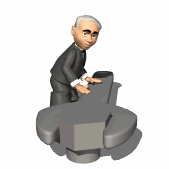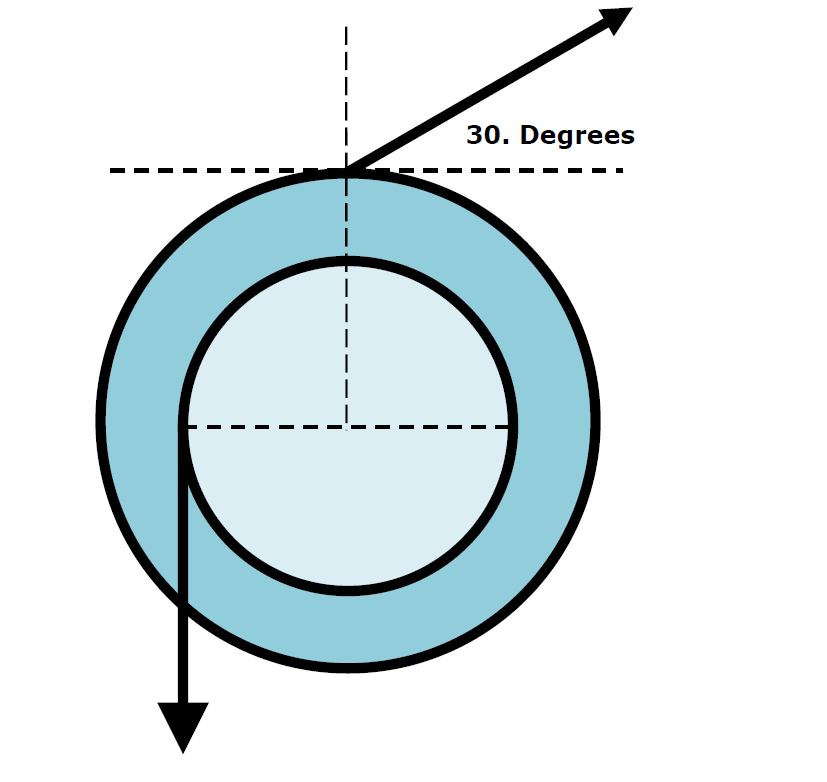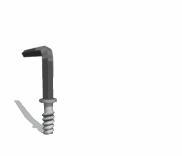HomeEx) Compound Wheel Find Net Torque, Both F =50. N r1 = 30 cm, r2 = 50 cmA 30 cm disk and a 50. cm disk are attached to an axle that passes through their centers. If a 50. N force hangs from the 30. cm disk and a  50. N force is applied to the 50. cm disk a shown above what is the net torque on the compound wheel CW  negative CCW positive   tnet = = .30 m(50. N) - .50 m(50. N)sin120°- 6.7 mNEx 2) A 2.20 m uniform beam with a mass of 25.0 kg has a 28.0 kg sign attached to it. Find all the forces on the beamFind the tension on the cable T and the components of wall's force on the beam  Fwally  Fwallx   I. Finding Cable Tension  St = 0TsinqL = mgL/2+MgL   Tsinq = mg/2+Mg   Tsin30 = mg/2+Mg   T/2 = mg/2+Mg   Ex 2) A 2.20 m uniform beam with a mass of 25.0 kg has a 28.0 kg sign attached to it.   T = mg + 2Mg  = 25.0 kg(9.8 m/s2)+ 2(28.0 kg)(9.8 m/s2)     T = 794 NII. Find Fwally & Fwallx       Force of beam on wall = Wall force on beam Tcosq = Fwallx    =794 Ncos30   Fwallx = 687 NII. Find FwallySFy = 0   mg + Mg = Fwally + Tsin30       Fwally = 123 N       Giancoli - p. 248) 18, 20, 21©Tony Mangiacapre., - All Rights Reserved [Home] Established 1995 Use any material on this site (w/ attribution)